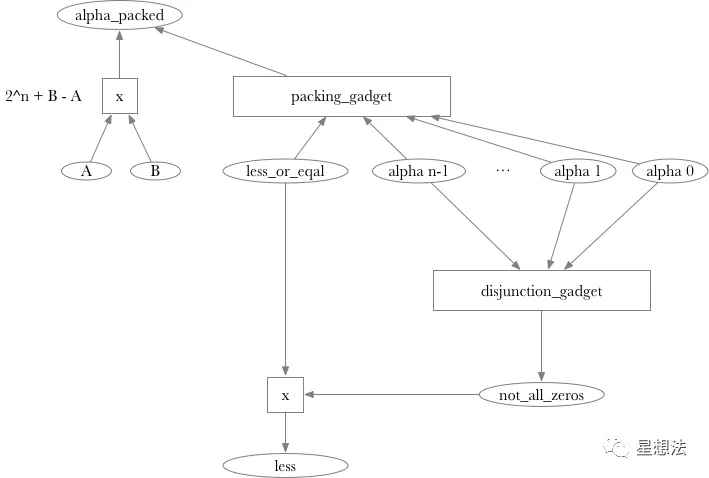# 零知识证明 – libsnark 源代码分析

•• 区块链资讯
2年前发布

libsnark 源代码，建议想深入零知识证明的小伙伴都读一读。Bellman 库主要围绕 Groth16 算法libsnark 给出了 SNARK 相关算法的全貌，各种 Relation，Language，Proof System。为了更好的生成 R1CS 电路，libsnark 抽象出 protoboard 和 gadget，方便开发者快速搭建电路。

``````commit 477c9dfd07b280e42369f82f89c08416319e24ae
Date:   Tue Jun 18 18:43:12 2019 -0400

Document that we also implement the Groth16 proof system.
``````

## 1. 源代码目录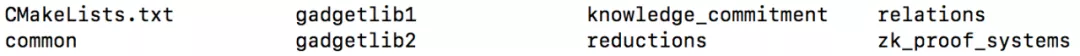common – 定义和实现了一些通用的数据结构，例如默克尔树，稀疏向量等等。

relations – relation 描述了 “约束” 关系。除了我们通常说的 R1CS 外，还有很多其他约束的描述语言。

reductions – 各种不同描述语言之间的转化。

knowledge_commit – 在 multiexp 的基础上，引入 pair 的概念，两个基点一个系数，计算结果称为一个 pair。

zk_proof_systems零知识证明中的各种证明系统（包括 Groth16，GM17 等等）。

## 2. Relation

libsnark 库总结了几种描述语言：

• constraint satisfaction problem 类
• R1CS – Rank-1 Constraint System
• USCS – Unitary-Square Constraint System
• circuit satisfaction problem 类
• BACS – Bilinear Arithmetic Circuit Satisfiability
• TBCS – Two-input Boolean Circuit Satisfiability
• ram computation 类RAM 是 Random Access Machine 的缩写。libsnark 总结了两种 RAM 计算框架：
• tinyRAM
• fooRAM
• arithmetic program 类
• QAP – Quadratic Arithmetic Program（GGPR13）
• SQP – Square Arithmetic Program（GM17）
• SSP – Square Span Program (DFGK14)

### 2.1 variable

varible 的定义非常简单，描述一个 variable，只需要记录一个 varible 对应的标号就行了。比如对应编号为 index 的 variable，表示的是 x_{index} 变量。

### 2.2 linear_term

linear_term 描述了一个线性组合中的一项。线性组合中的一项由变量以及对应的系数组成：

### 2.3linear_combination

linear_combination 描述了一个完整的线性组合。一个 linear combination 由多个 linear term 组成：

### 2.4R1CS

R1CS 定义在 constraint_satisfaction_problems/r1cs/r1cs.hpp。R1CS 约束就是满足以下形式的一个表达式：

`< A , X > * < B , X > = < C , X >`

`X` 是所有变量组合的向量，`A/B/C` 是和 X 等长的向量。`<,>` 代表的是点乘。一个 R1CS 系统由多个 R1CS 约束组成。

R1CS 约束定义为：

### 2.5QAP

QAP 定义在 arithmetic_programs/qap/qap.hpp。libsnark 采用的 QAP 的公式是：`A*B-C=H*Z`

numbariables 表示 QAP 电路的变量的个数。numinputs 表示 QAP 电路的”statement” 对应变量的个数。degree_表示 A/B/C 中每个多项式的阶的个数（和电路的门的个数相关）。

domain 是计算傅立叶变换 / 反傅立叶变换的引擎，由 libfqfft 库实现。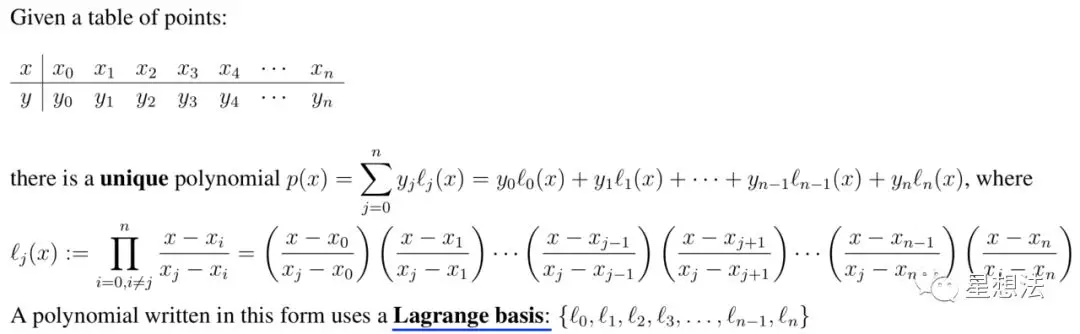A_in_Lagrange_basis/B_in_Lagrange_basis/C_in_Lagrange_basis 把一个电路中每个变量不同门的值整理在一起。举个例子，如下是 x^3+x+5 的电路对应的 R1CS 的约束：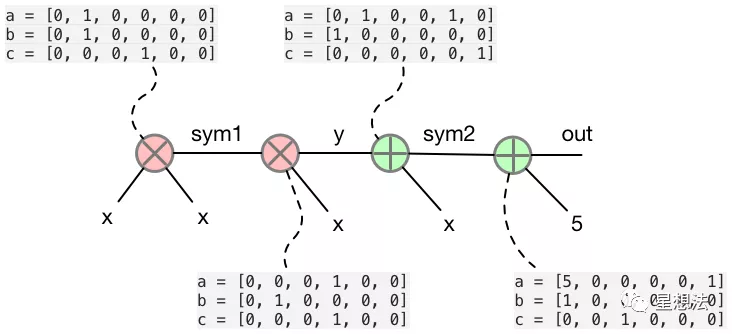qap_instance 描述的是一个 QAP 电路，A/B/C 对应的多项式表达式（虽然是用 Lagrange basis 表示）。A/B/C 多项式在一个点上的结果，用 qap_instance_evaluation 表示：

qap_instance_evaluation，记录了在 t 点上，A/B/C/H 以及 Z 对应的值。

coefficients_for_ABCs 就是 witness。为了计算的方便，同时给出了对应的 H 多项式的系数。 在给定一个 qap_instance_evaluation 和一个 qap_witness 的前提下，可以通过 is_satisfied 函数确定，是否 witness 合理：

## 3. Reduction

Reduction 实现了不同描述语言之间的转化。libsnark 给出了如下一系列的转化实现：

• bacs -> r1cs
• r1cs -> qap
• r1cs -> sap
• ram -> r1cs
• tbcs -> uscs
• uscs -> ssp

### 3.1 r1cs_to_qap_instance_map

r1cs_to_qap_instance_map 函数实现了从一个 R1CS 实例到 QAP instance 的转化。转化过程比较简单，就是将系数重新整理的过程（可以查看 2.5 中的 QAP 的描述）。

### 3.2 r1cs_to_qap_instance_map_with_evaluation

r1cs_to_qap_instance_map_with_evaluation 函数实现了从一个 R1CS 实例到 qap_instance_evaluation 的转化。给定 t，计算 A/B/C 以及 H/Z。

### 3.3 r1cs_to_qap_witness_map

r1cs_to_qap_witness_map 函数实现了从一个 R1CS 实例到 qap_witness 的转化。

H 多项式系数的计算相对复杂一些，涉及到傅立叶变换 / 反傅立叶变换。H 多项式的计算公式计算如下： `H(z) := (A(z)*B(z)-C(z))/Z(z)`

1. 确定 A 和 B 的多项式（通过反傅立叶变换）
1. 计算 A 和 B，对应 FieldT::multiplicative_generator 的计算结果
2. 确定 C 的多项式（通过反傅立叶变换）
3. 计算 C，对应 FieldT::multiplicative_generator 的计算结果
1. 计算 H，对应 FieldT::multiplicative_generator 的计算结果
2. 计算 H 多项式的系数（反傅立叶变换）

## 4. ZK Proof System

libsnark 提供了四种证明系统：

• pcd (Proof-Carrying Data)
• ppzkadsnark (PreProcessing Zero-Knowledge Succinct Non-interactive ARgument of Knowledge Over Authenticated Data)
• ppzksnark (PreProcessing Zero-Knowledge Succinct Non-interactive ARgument of Knowledge)
• zksnark (Zero-Knowledge Succinct Non-interactive ARgument of Knowledge)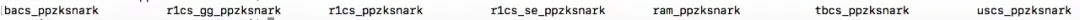r1cs_gg_ppzksnark，gg 代表 General Group，就是 Groth16 算法。

r1cs_se_ppzksnark，se 代表 Simulation Extractable，就是 GM17 算法。

r1cs_ppzksnark，就是 PGHR13 算法。

### 4.1 r1cs_gg_ppzksnark_proving_key

r1cs_gg_ppzksnark_proving_key 记录了 CRS 中在 prove 过程需要的信息。

A_query 是 A (t) 以 G1 生成元的 multiexp 的计算结果。

B_query 是 B (t) 以 G1/G2 生成元的 multiexp 的计算结果。

H_query 是如下的计算以 G1 位生成元的 multiexp 的计算结果：

`H(t)*Z(t)/delta`

L_query 是如下的计算在 G1 位生成元的 multiexp 的计算结果：

`(beta*A(t)+alpha*B(t)+C(t))/delta`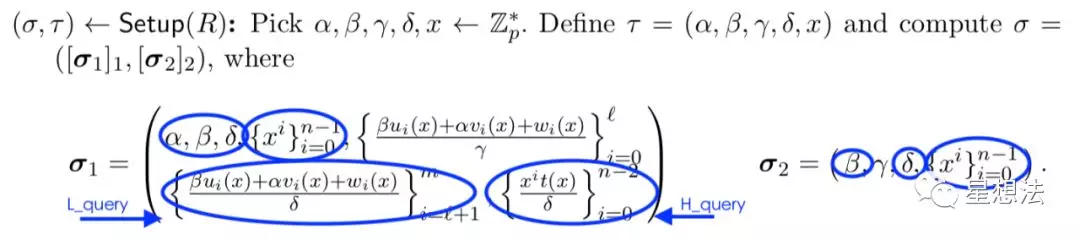`r1cs_gg_ppzksnark_constraint_system<ppT>` 定义在 zk_proof_systems/ppzksnark/r1cs_gg_ppzksnark/r1cs_gg_ppzksnark_params.hpp 文件中。

### 4.2 r1cs_gg_ppzksnark_verification_key

r1cs_gg_ppzksnark_verification_key 记录了 CRS 中在 verify 过程需要的信息。### 4.3 r1cs_gg_ppzksnark_processed_verification_key

r1cs_gg_ppzksnark_processed_verification_key 和 r1cs_gg_ppzksnark_verification_key 类似。“processed” 意味着 verification key 会做进一步处理，验证的过程会更快。

### 4.4 r1cs_gg_ppzksnark_keypair

r1cs_gg_ppzksnark_keypair 包括两部分：r1cs_gg_ppzksnark_proving_key 和 r1cs_gg_ppzksnark_verification_key。

### 4.6 r1cs_gg_ppzksnark_generator

r1cs_gg_ppzksnark_generator 给定一个 r1cs_constraint_system 的基础上，r1cs_gg_ppzksnark_generator 能生成 r1cs_gg_ppzksnark_keypair，也就是生成 CRS 信息。

### 4.8 r1cs_gg_ppzksnark_verifier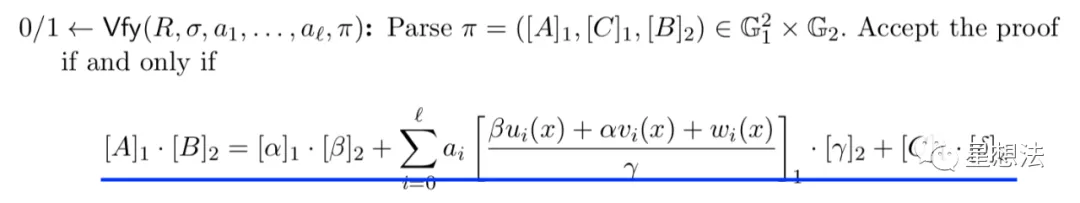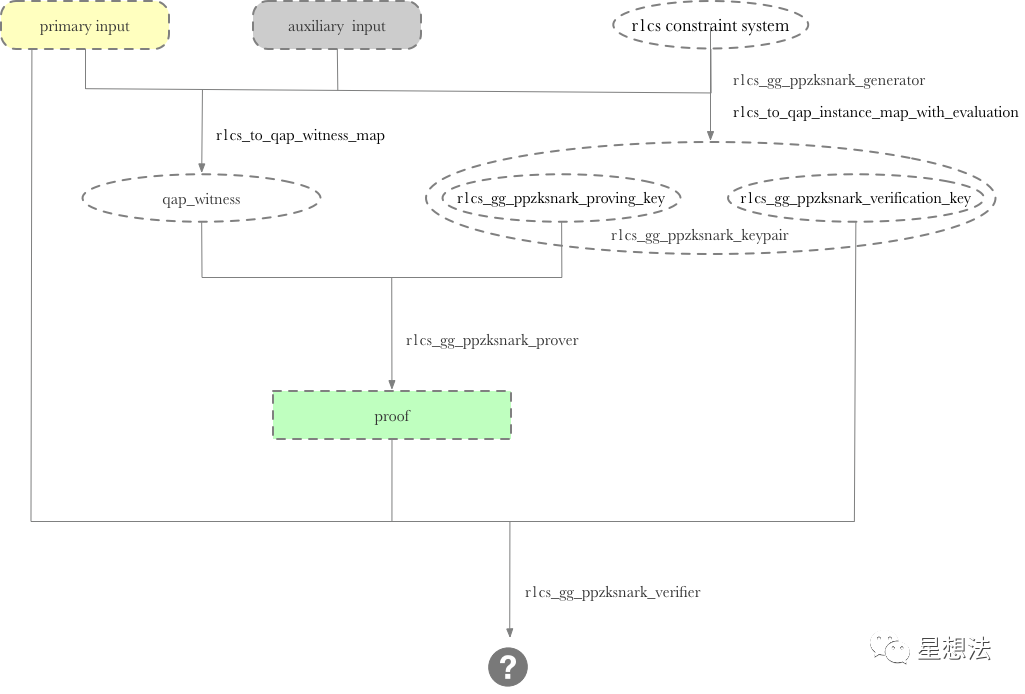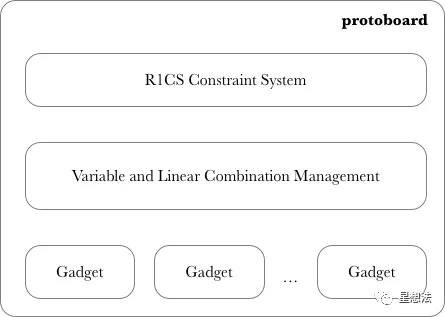### 5.1 protoboard

protoboard 是 r1cs_constraint_system 之上的一层封装。通过一个个的 Gadget，向 r1cs_constraint_system 添加约束。为了让不同的 Gadget 之间采用统一的 Variable 以及 Lc，protoboard 通过”next_free_var” 以及”next_free_lc“维护所有 Gadget 创建的 Variable 以及 Lc。

### 5.2 pb_variable

libsnark 提供了在 pb_variable，pb_variable_array，pb_linear_combination 和 pb_linear_combination_array 四个类。这四个类都是 variable, linear_combination 的封装，为了支持 protoboard 的管理。

1. 申请 Variable 或者 Lc （allocate）
3. 生成相关的 Witness（generate_r1cs_witness）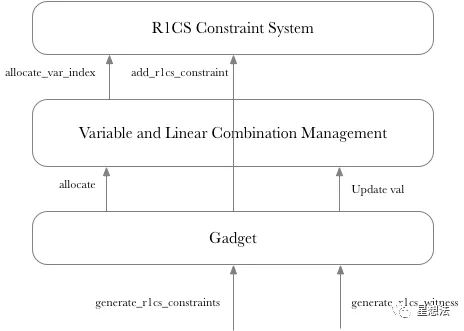### 5.4 example

comparison_gadget 的构造函数比较清晰：在给定两个 n 位的数 A 和 B，输出两个变量：less 和 less_or_eq（A 是否小于 B？）。

alpha 是 2^n+B-A 的结果的位的表示。alpha_packed 是一个变量，alpha 对应的值。也就是，alpha_packed 等于 2^n+B-A。

not_all_zeros 是一个变量，表示 B-A 的结果是否全是 0。

all_zeros_test 是 disjunction_gadget，确定 alpha 的 n 个变量中是否全 0。

a. 对 not_all_zeros，添加 boolean 约束（该变量只能是 0 或者 1）

b. pack_alpha->generate_r1cs_constraints (true) 约束 alpha 对应的数值等于 alpha_packed。

c. 1*(2^n+B-A) = alpah_packed

d. 确定 not_all_zeros 变量的值和 alpha 中 n 个变量中是否为 0 的结果一致

e. less_or_eq * not_all_zeros = less